# Maharashtra Board 11th Maths Solutions Chapter 8 Measures of Dispersion Ex 8.2

Balbharti Maharashtra State Board Class 11 Maths Solutions Pdf Chapter 8 Measures of Dispersion Ex 8.2 Questions and Answers.

## Maharashtra State Board 11th Maths Solutions Chapter 8 Measures of Dispersion Ex 8.2

Question 1.
Find variance and S.D. for the following set of numbers.
7, 11, 2, 4, 9, 6, 3, 7, 11, 2, 5, 8, 3, 6, 8, 8, 2, 6
Solution:
Given data:
7, 11, 2, 4, 9, 6, 3, 7, 11, 2, 5, 8, 3, 6, 8, 8, 2, 6
The tabulated form of the above data is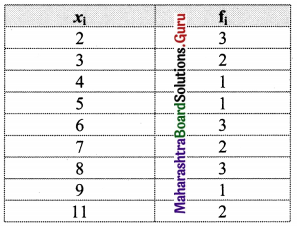Calculation of variance and S.D.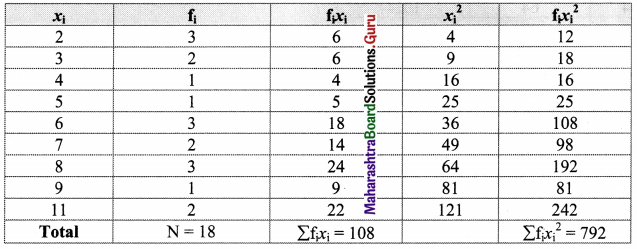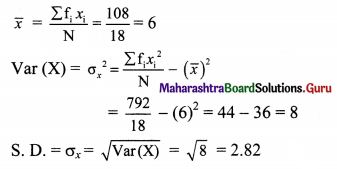Question 2.
Find variance and S.D. for the following set of numbers.
65, 77, 81, 98, 100, 80, 129
Solution: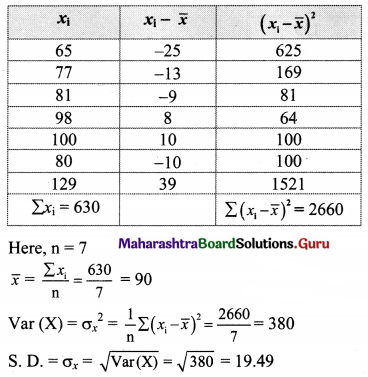Question 3.
Compute variance and standard deviation for the following data: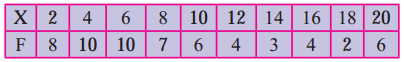Solution: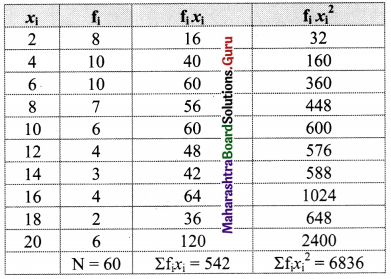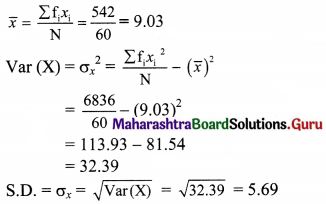Question 4.
Compute the variance and S.D.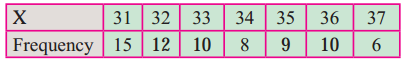Solution:
Let u = $$\frac{x-\mathrm{A}}{\mathrm{h}}=\frac{x-34}{1}$$
Calculation of variance of u: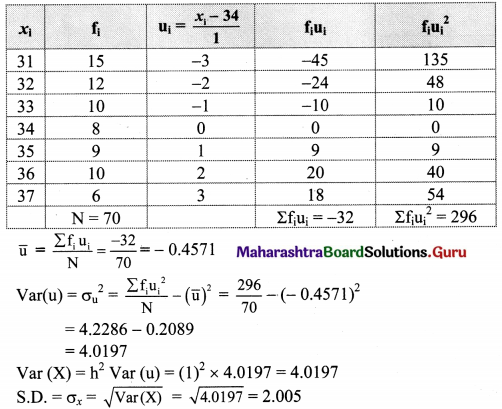Question 5.
Following data gives ages of 100 students in a college. Calculate variance and S.D.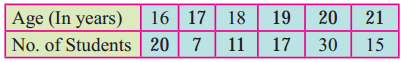Solution:
Let u = $$\frac{x-\mathrm{A}}{\mathrm{h}}=\frac{x-19}{1}$$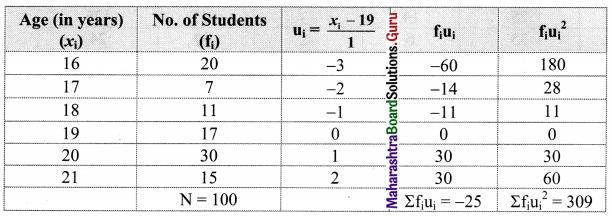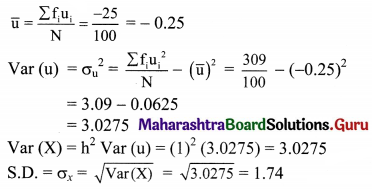Question 6.
Find mean, variance and S.D. of the following data.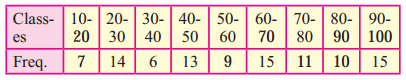Solution: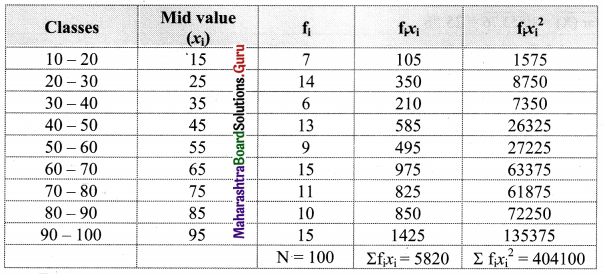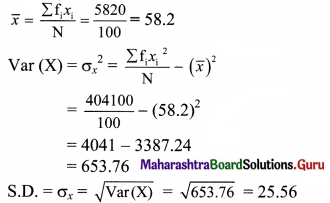Alternate Method:
Let u = $$\frac{x-\mathrm{A}}{\mathrm{h}}=\frac{x-55}{10}$$
Calculation of variance of u: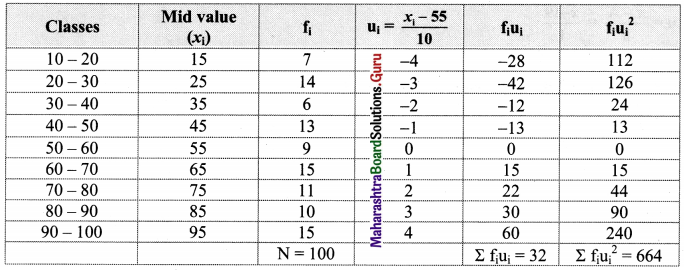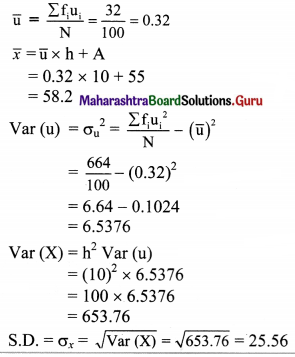Question 7.
Find the variance and S.D. of the following frequency distribution which gives the distribution of 200 plants according to their height.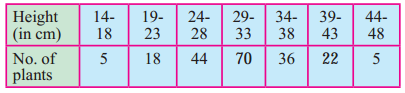Solution:
Since data is not continuous, we have to make it continuous.
Let u = $$\frac{x-\mathrm{A}}{\mathrm{h}}=\frac{x-31}{5}$$
Calculation of variance of u: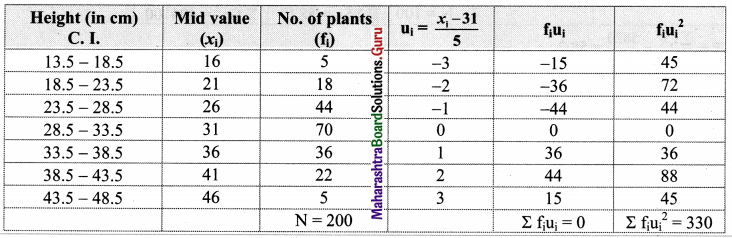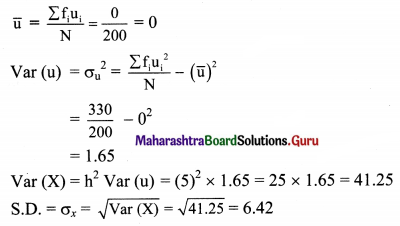Question 8.
The mean of 5 observations is 4.8 and the variance is 6.56. If three of the five observations are 1, 3, and 8, find the other two observations.
Solution:
$$\bar{x}$$ = 4.8, Var (X) = 6.56, n = 5, x1 = 1, x2 = 3, x3 = 8 ……(given)
Let the remaining two observations be x4 and x5.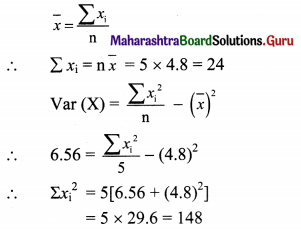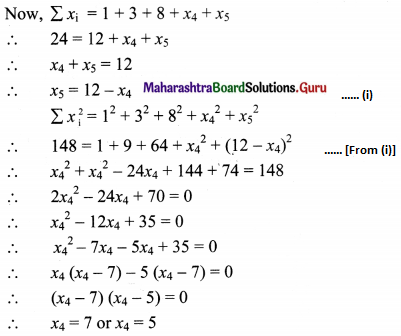From (i), we get
x5 = 5 or x5 = 7
∴ The two numbers are 5 and 7.